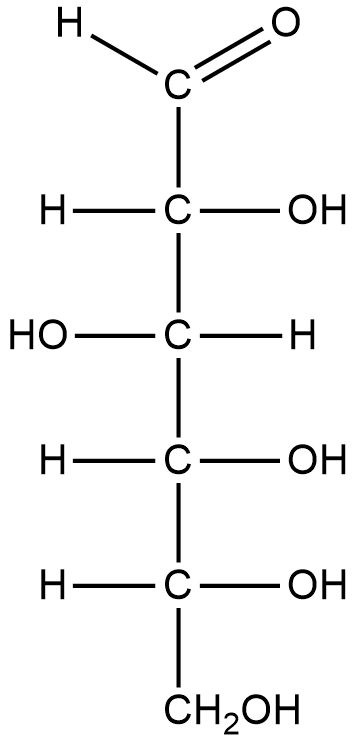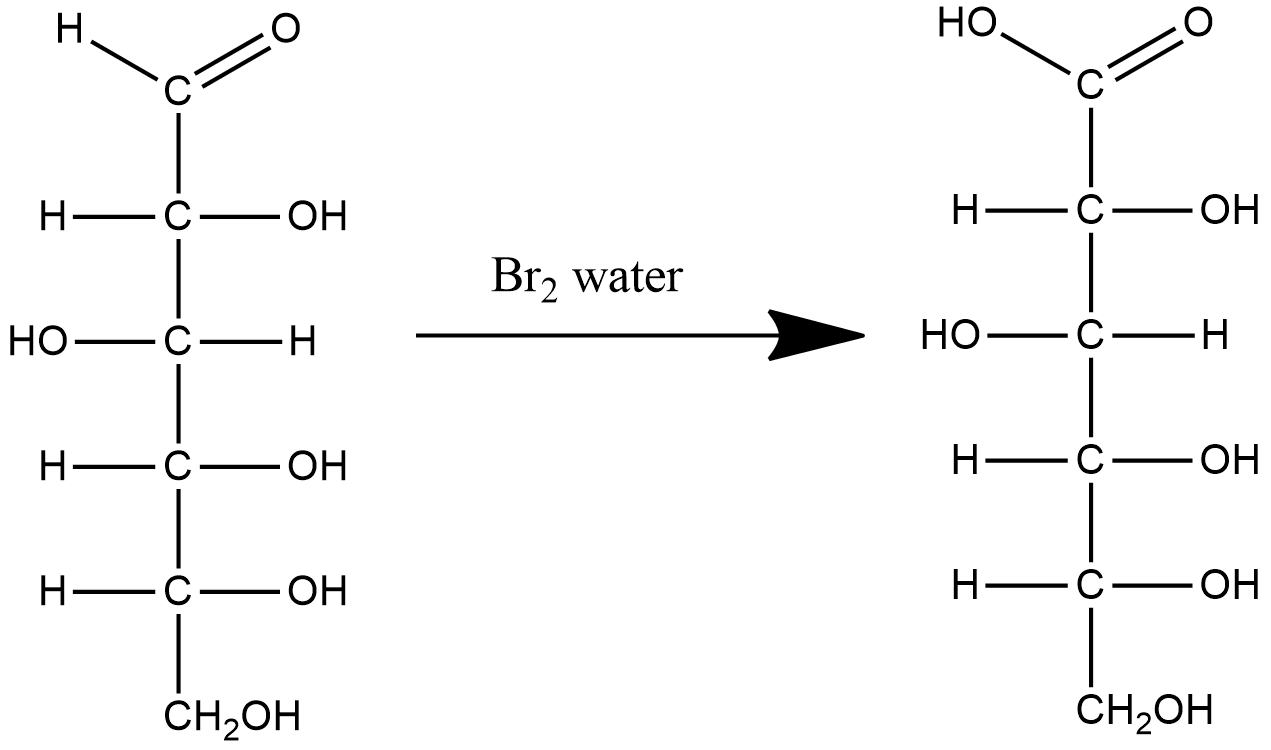QuestionAnswers

# Glucose on reaction with $B{{r}_{2}}$ water gives:

Hint: The Fischer projection of glucose molecule reveals that there is an aldehyde group at one of the ends which can be easily oxidized. On the other hand, $B{{r}_{2}}$water is considered as a mild oxidizing agent that can oxidize aldehyde groups to carboxylic acid.

Glucose is the most abundant monosaccharide, a subcategory of carbohydrates. Glucose is mainly made by plants and most algae during photosynthesis from water and carbon dioxide, using energy from sunlight, where it is used to make cellulose in cell walls, which is the most abundant carbohydrate.
Let us draw the structure of glucose to see the functional groups present in the molecule
Fischer projection of glucose:From the above structure we can identify 5 hydroxyl groups and 1 aldehyde group.
$B{{r}_{2}}$ water is widely used to detect unsaturation in a compound. The discoloration of $B{{r}_{2}}$ water indicates unsaturation in the compound.
However, $B{{r}_{2}}$ water is also considered as a mild oxidizing agent. $B{{r}_{2}}$ water oxidizes aldehydes into carboxylic acids. They do not react with ketone due to steric hindrance.
Let us write down the reaction of glucose molecules with $B{{r}_{2}}$ water.In the above reaction we see that $B{{r}_{2}}$ water oxidizes the aldehyde group present in glucose to carboxylic acid. The product of this reaction is gluconic acid.

Therefore, the correct answer is option (B).

Note: Glucose when subjected to a strong oxidizing agent, gives saccharic acid the product which has a carboxylic acid group on each end of the molecule. The terminal hydroxyl group does not get oxidized as $B{{r}_{2}}$ water is a mild oxidizing agent and cannot oxidize hydroxyl groups.